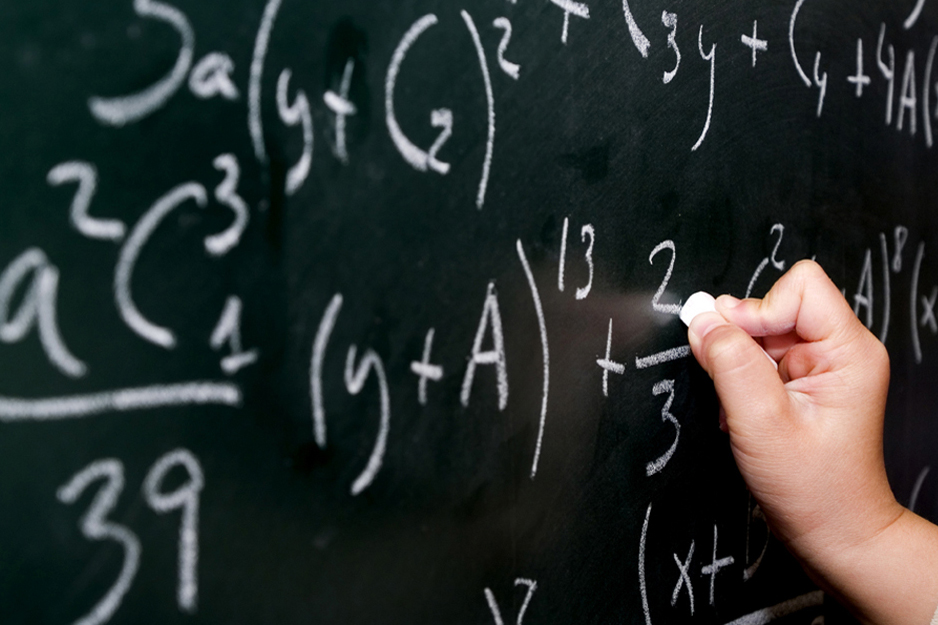# Mathematics### Mathematics – Course code 9709

The course aims to develop a deeper understanding of mathematical principles in pupils;  the further development of mathematical skills including the use of applications of mathematics in the context of everyday situations and in other subjects that they may be studying; the ability to analyze problems logically, recognizing when and how a situation may be represented mathematically;  the use of mathematics as a means of communication;  a solid foundation for further study.
AS Level candidates take

 Paper 1: Pure Mathematics 1 (P1) 1¾ hours About 10 shorter and longer questions 75 marks weighted at 60% of total

And any ONE of the following

 Paper 2: Pure Mathematics 2 (P2) Paper 4: Mechanics 1 (M1) Paper 6: Probability and Statistics 1 (S1) 1¼ hours About 7 shorter and longer questions 50 marks weighted at 40% of total 1¼ hours About 7 shorter and longer questions 50 marks weighted at 40% of total 1¼ hours About 7 shorter and longer questions 50 marks weighted at 40% of total

A Level candidates take

 Paper 1: Pure Mathematics 1 (P1) Paper 3 Pure Mathematics 3 (P3) 1¾ hours About 10 shorter and longer questions 75 marks weighted at 30% of total 1¾ hours About 10 shorter and longer questions 75 marks weighted at 30% of total

And any ONE of the following combinations

 Paper 4: Mechanics 1 (M1) Paper 6: Probability and Statistics 1 (S1) 1¼ hours About 7 shorter and longer questions 50 marks weighted at 20% of total 1¼ hours About 7 shorter and longer questions 50 marks weighted at 20% of total